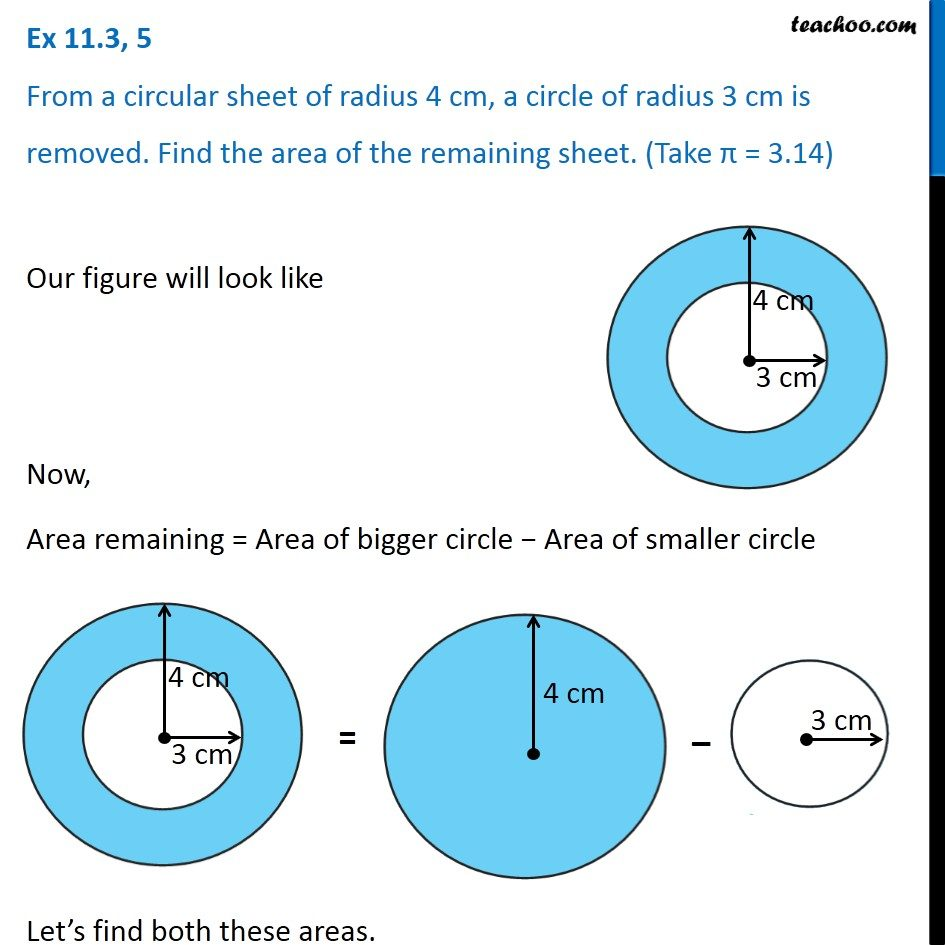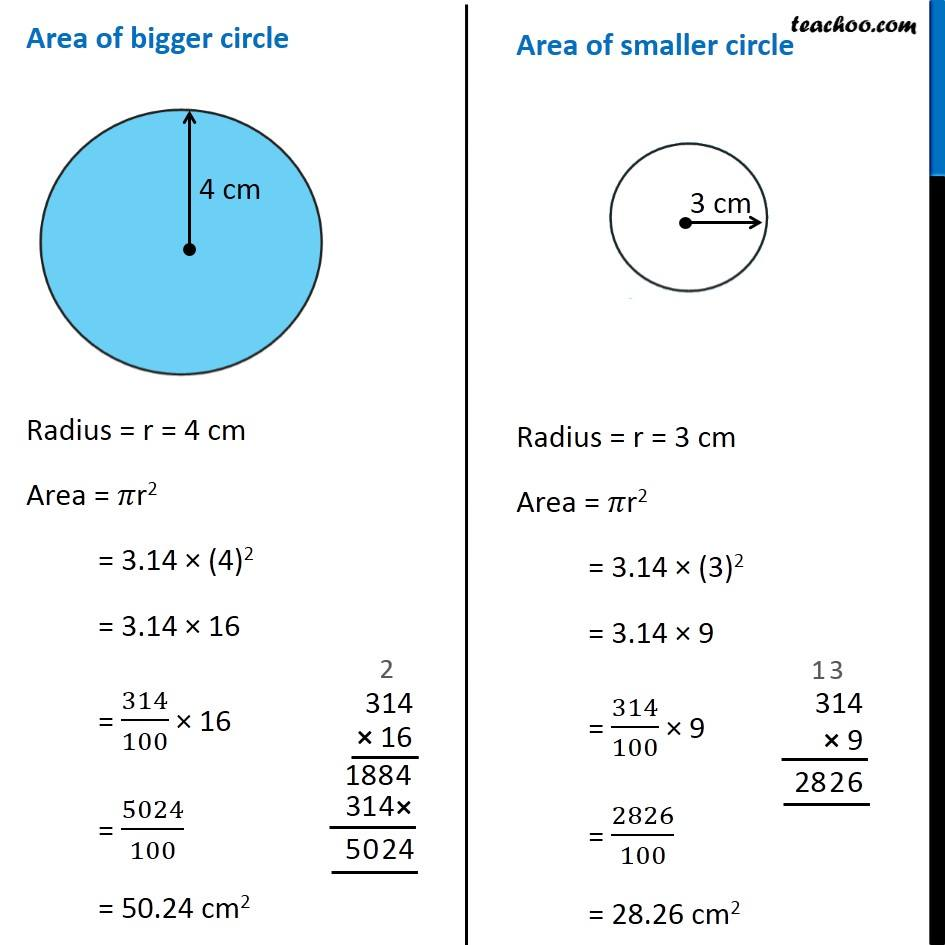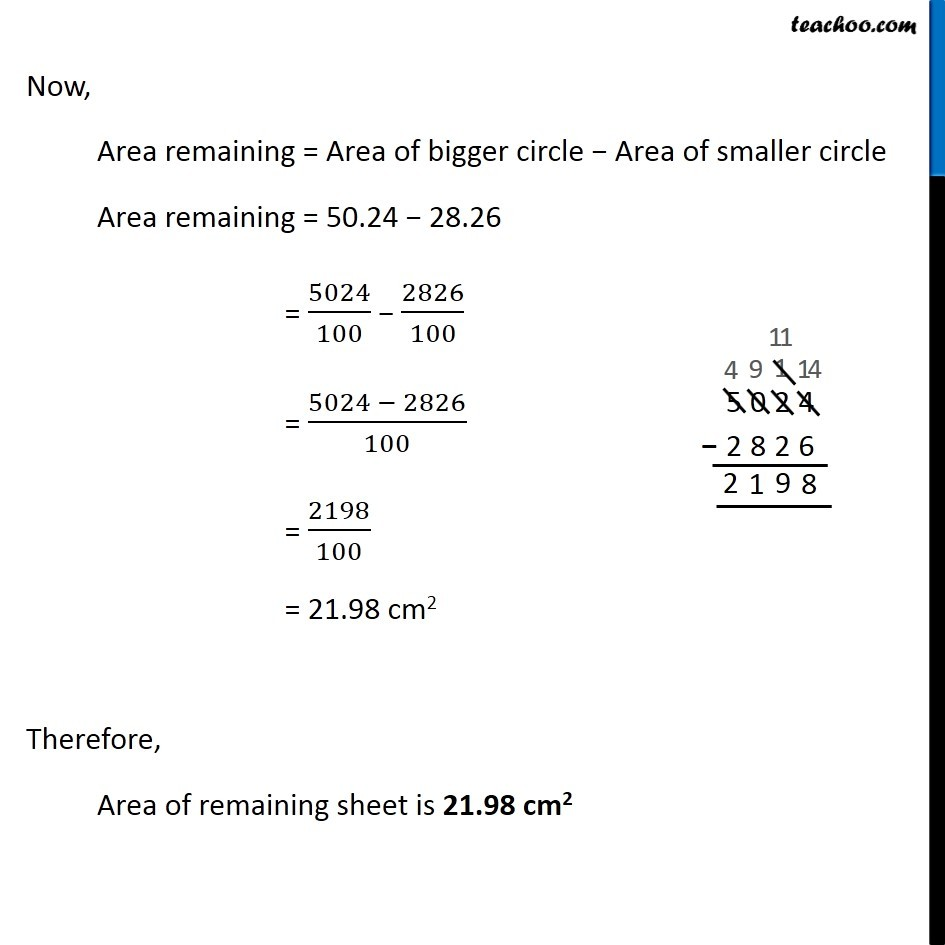Area of combination of figures - two circles, circle and square

Chapter 9 Class 7 Perimeter and Area
Concept wiseGet live Maths 1-on-1 Classs - Class 6 to 12

### Transcript

Ex 9.2, 5 From a circular sheet of radius 4 cm, a circle of radius 3 cm is removed. Find the area of the remaining sheet. (Take π = 3.14) Our figure will look like Now, Area remaining = Area of bigger circle − Area of smaller circle Let’s find both these areas. Area of bigger circle Radius = r = 4 cm Area = 𝜋r2 = 3.14 × (4)2 = 3.14 × 16 = 314/100 × 16 = 5024/100 = 50.24 cm2 Area of smaller circle Radius = r = 3 cm Area = 𝜋r2 = 3.14 × (3)2 = 3.14 × 9 = 314/100 × 9 = 2826/100 = 28.26 cm2 Now, Area remaining = Area of bigger circle − Area of smaller circle Area remaining = 50.24 − 28.26 = 5024/100 − 2826/100 = (5024 − 2826)/100 = 2198/100 = 21.98 cm2 Therefore, Area of remaining sheet is 21.98 cm2